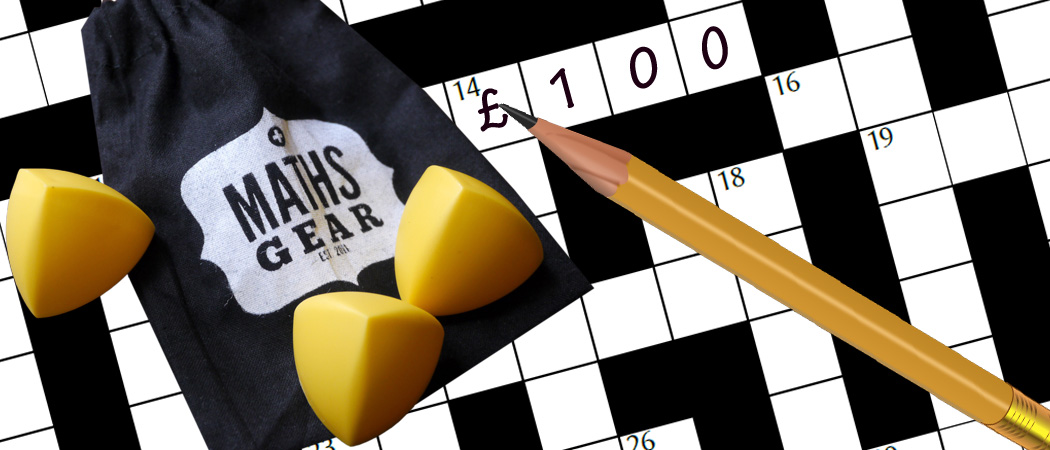# Prize crossnumber, Issue 07

Win £100 of Maths Gear goodies by solving our famously fiendish crossnumberOur original prize crossnumber is featured on pages 52 and 53 of Issue 07.

Clarification: Added “non-zero” to clues 10A, 19D.
Clarification: For 20D, 0 is not a factor of any number, so the number contains no 0s.

### Rules

• Although many of the clues have multiple answers, there is only one solution to the completed crossnumber. As usual, no numbers begin with 0. Use of Python, OEIS, Wikipedia, etc. is advised for some of the clues.
• One randomly selected correct answer will win a £100 Maths Gear goody bag. Three randomly selected runners up will win a Chalkdust t-shirt. The prizes have been provided by Maths Gear, a website that sells nerdy things worldwide, with free UK shipping. Find out more at mathsgear.co.uk
• To enter, submit the sum of the across clues via this form by 1 August 2018. Only one entry per person will be accepted. Winners will be notified by email and announced on our blog by 19 August 2018.

### Crossnumber

Crossnumber #7, set by Humbug:

### Clues

#### Across

• 1. The first digit of this number is equal to the last digit of 34A. (16)
• 9. The product of the digits of 30A. Also the sum of the digits of 1A. (2)
• 10. The product of this number’s digits is a (non-zero) multiple of 1,000,000. (14)
• 13. None of the digits of 34A is equal to the middle digit of this number. (3)
• 14. All the digits of this number except one are the same. (10)
• 17. The sum of two positive cube numbers. (3)
• 18. The product of the digits of 16D. (6)
• 21. All the digits of this number except one are the same. (5)
• 22. Greater than 13A. (3)
• 23. The product of the digits of 6D. (4)
• 25. None of this number’s digits are 0, and the sum of this number’s digits is greater than the product of its digits. (4)
• 26. A number $n$ such that the last three digits of $2^n$ are equal to $n$. (3)
• 27. One more than the product of the digits of 23A. (3)
• 28. A multiple of 9. (10)
• 30. The product of the digits of one less than 27A. (3)
• 31. The product of this number’s digits is 7D. (12)
• 32. The sum of the digits of 34A. (2)
• 34. The product of this number and 1A is a multiple of 1,000,000,000,000. (16)

#### Down

• 2. This number is equal to the sum of the cubes of its digits. (3)
• 3. The first three digits of this number are all even. (14)
• 4. The sum of the cubes of the digits of 5D. (3)
• 5. This number is not equal to 4D, but it is equal to the sum of the cubes of the digits of 4D. (3)
• 6. The product of the digits of 18A. (5)
• 7. A triangle number whose digits add to 18. (3)
• 8. A power of 13A. (16)
• 10. A power of 22A. (14)
• 11. This number is equal to the sum of the cubes of its digits. (3)
• 12. A power of 13A. (14)
• 15. Equal to the last 8 digits of 28A. (8)
• 16. The product of the digits of 3D. (12)
• 19. The product of this number’s digits is a (non-zero) multiple of 9. (4)
• 20. Each digit of this number is a factor of the previous digit. (6)
• 24. This number is equal to the sum of the fourth powers of its digits. (4)
• 29. This number is equal to the sum of the fifth powers of its digits. (5)
• 33. The product of the digits of 9A. (2)